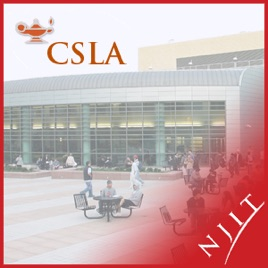1 episode

Some of the topics that are covered include:
A Brief Review of Functions.
Introduction To and Definition of Limits, Calculation of Limits Using Limit Laws, Asymptotes, Velocities and Tangents.
Definition of Derivative, Calculation of Derivatives of Common Functions, Rules for Differentiation, Implicit Differentiation.
Application of Derivatives: Related Rates, Linear Approximation, Finding Extrema, Curve Sketching, Mean Value Theorem, Evaluating Limits of Indeterminant Forms/L'Hospital's Rule, Optimization, Rootfinding.
Antiderivatives, Integration, Fundamental Theorem of Calculus, Substitution Rule# MATH 111: Calculus I Honors - Bukiet - Recovered Trash NJIT

• Podcasts

Some of the topics that are covered include:
A Brief Review of Functions.
Introduction To and Definition of Limits, Calculation of Limits Using Limit Laws, Asymptotes, Velocities and Tangents.
Definition of Derivative, Calculation of Derivatives of Common Functions, Rules for Differentiation, Implicit Differentiation.
Application of Derivatives: Related Rates, Linear Approximation, Finding Extrema, Curve Sketching, Mean Value Theorem, Evaluating Limits of Indeterminant Forms/L'Hospital's Rule, Optimization, Rootfinding.
Antiderivatives, Integration, Fundamental Theorem of Calculus, Substitution Rule

• video
ReviewofWhatYouShouldKnow

• 5 min